# Introduction to Trees

## Introduction

Lists, stacks, and queues, are all linear structures: in all three data structures, one item follows another. Trees will be our first non-linear structure:

• More than one item can follow another.
• The number of items that follow can vary from one item to another.

Trees have many uses:

• representing family genealogies
• as the underlying structure in decision-making algorithms
• to represent priority queues (a special kind of tree called a heap)
• to provide fast access to information in a database (a special kind of tree called a b-tree)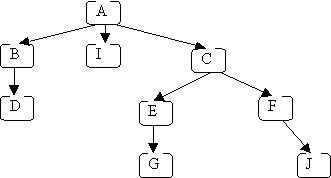So a (computer science) tree is kind of like an upside-down real tree...

A path in a tree is a sequence of (zero or more) connected nodes; for example, here are 3 of the paths in the tree shown above:

`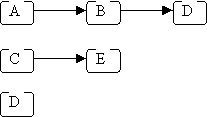`

The length of a path is the number of nodes in the path, e.g.:

```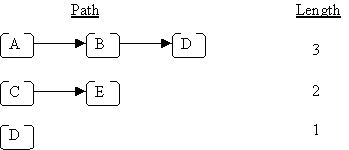```

The height of a tree is the length of the longest path from the root to a leaf; for the above example, the height is 4 (because the longest path from the root to a leaf is A → C → E → G, or A → C → E → J). An empty tree has height = 0.

The depth of a node is the length of the path from the root to that node; for the above example:

• the depth of J is 4
• the depth of D is 3
• the depth of A is 1

Given two connected nodes like this:

`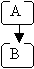`

Node A is called the parent, and node B is called the child.

A subtree of a given node includes one of its children and all of that child's descendants. The descendants of a node n are all nodes reachable from n (n's children, its children's children, etc.). In the original example, node A has three subtrees:

1. B, D
2. I
3. C, E, F, G, J.

An important special kind of tree is the binary tree. In a binary tree:

• Each node has 0, 1, or 2 children.
• Each child is either a left child or a right child.

### Representing Trees

Since a binary-tree node never has more than two children, a node can be represented using a class with 3 fields: one for the data in the node, plus two child pointers:

```class BinaryTreenode {
// *** fields ***
private Object data;
private BinaryTreenode leftChild;
private BinaryTreenode rightChild;
}```

However, since a general-tree node can have an arbitrary number of children, a fixed number of child-pointer fields won't work. Instead, we can use a List to keep all of the child pointers:

```class Treenode {
// *** fields ***
private Object data;
private List children;
}```
(Note that the items in the List will be of type Treenode.)

As we know, a list can be represented using either an array or a linked-list. For example, consider this general tree (a simplified version of the original example):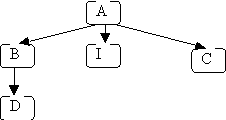For the array representation of the List (where the array has an initial size of 4) we would have: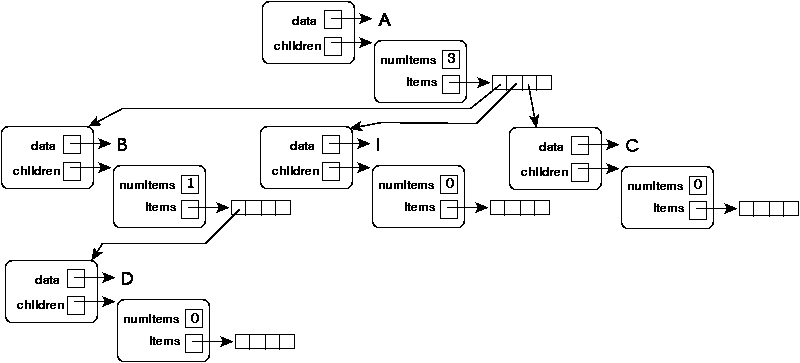TEST YOURSELF #1

Draw a similar picture of the tree when the List fields are implemented using linked lists.

### Tree Traversals

It is often useful to iterate through the nodes in a tree:

• to print all values
• to determine if there is a node with some property
• to make a copy

When we iterated through a List, we started with the first node and visited each node in turn. Since each node is visited, the best possible complexity is O(N) for a tree with N nodes. All of our traversal methods will achieve this complexity.

`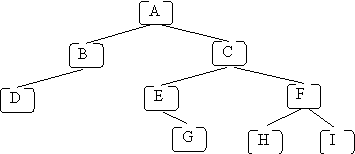`

Preorder

A preorder traversal can be defined (recursively) as follows:

1. visit the root
2. perform a preorder traversal of the first subtree of the root
3. perform a preorder traversal of the second subtree of the root
4. etc. for all the subtrees of the root

If we use a preorder traversal on the example tree given above, and we print the letter in each node when we visit that node, the following will be printed: A B D C E G F H I.

Postorder

A postorder traversal is similar to a preorder traversal, except that the root of each subtree is visited last rather than first:

1. perform a postorder traversal of the first subtree of the root
2. perform a postorder traversal of the second subtree of the root
3. etc. for all the subtrees of the root
4. visit the root

If we use a postorder traversal on the example tree given above, and we print the letter in each node when we visit that node, the following will be printed: D B G E H I F C A.

Level order

The idea of a level-order traversal is to visit the root, then visit all nodes "1 level away" (depth 2) from the root (left to right), then all nodes "2 levels away" (depth 3) from the root, etc. For the example tree, the goal is to visit the nodes in the following order:

`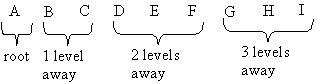`

A level-order traversal requires using a queue (rather than a recursive algorithm, which implicitly uses a stack). Here's how to print the data in a tree in level order, using a queue Q, and using an iterator to access the children of each node (we assume that the root node is called root, and that the Treenode class provides a getChildren method):

```Q.enqueue(root)
while (!Q.empty()) {
Treenode n = Q.dequeue();
System.out.print(n.getData());
List L = n.getChildren();
Iterator it = L.iterator();
while (it.hasNext()) {
Q.enqueue(it.next());
}
}```

TEST YOURSELF #2

Draw pictures of Q as it would be each time around the outer while loop in the code given above for the example tree given above.

In-order

An in-order traversal involves visiting the root "in between" visiting its left and right subtrees. Therefore, an in-order traversal only makes sense for binary trees. The (recursive) definition is:

1. perform an in-order traversal of the left subtree of the root
2. visit the root
3. perform an in-order traversal of the right subtree of the root

If we print the letters in the nodes of our example tree using an in-order traversal, the following will be printed: D B A E G C H F I

The primary difference between the preorder, postorder and in-order traversals is where the node is visited in relation to the recursive calls; i.e., before, after or in-between.

What is printed when the following tree is visited using (a) a preorder traversal, (b) a postorder traversal, (c) a level-order traversal, and (d) an in-order traversal?

`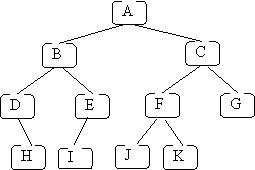`